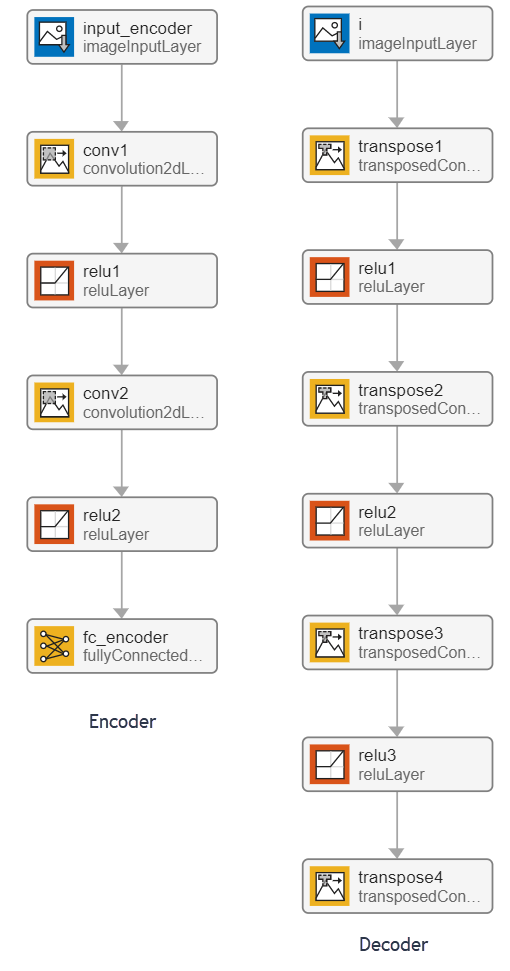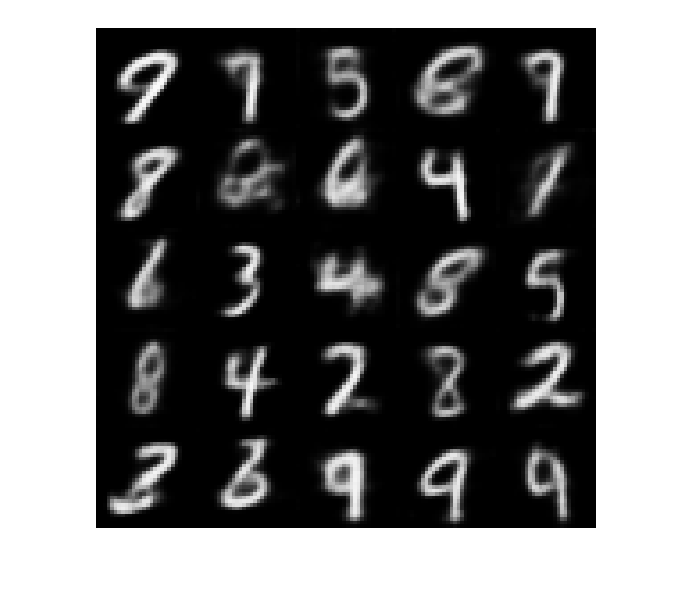# Generate Digit Images on NVIDIA GPU Using Variational Autoencoder

This example shows how to generate CUDA® MEX for a trained variational autoencoder (VAE) network. The example illustrates:

• Generation of hand-drawn digit images in the style of the MNIST data set.

• CUDA code generation for a `dlnetwork` (Deep Learning Toolbox) object representing a deep learning network.

• Use of `dlarray` (Deep Learning Toolbox) objects in code generation.

This example uses a pretrained decoder network based on the Train Variational Autoencoder (VAE) to Generate Images example from the Deep Learning Toolbox™. For more information, see Train Variational Autoencoder (VAE) to Generate Images (Deep Learning Toolbox).

### Third-Party Prerequisites

#### Required

• CUDA enabled NVIDIA® GPU and compatible driver.

#### Optional

For non-MEX builds such as static, dynamic libraries or executables, this example has the following additional requirements.

### Verify GPU Environment

To verify that the compilers and libraries for running this example are set up correctly, use the `coder.checkGpuInstall` function.

```envCfg = coder.gpuEnvConfig('host'); envCfg.DeepLibTarget = 'cudnn'; envCfg.DeepCodegen = 1; envCfg.Quiet = 1; coder.checkGpuInstall(envCfg);```

### Pretrained Variational Autoencoder Network

Autoencoders have two parts: the encoder and the decoder. The encoder takes an image input and outputs a compressed representation (the encoding), which is a vector of size `latent_dim`, equal to 20 in this example. The decoder takes the compressed representation, decodes it, and recreates the original image.

VAEs differ from regular autoencoders in that they do not use the encoding-decoding process to reconstruct an input. Instead, they impose a probability distribution on the latent space, and learn the distribution so that the distribution of outputs from the decoder matches that of the observed data. Then, they sample from this distribution to generate new data.

This example uses the decoder network trained in the Train Variational Autoencoder (VAE) to Generate Images example. To train the network yourself, see Train Variational Autoencoder (VAE) to Generate Images (Deep Learning Toolbox).### The generateVAE Entry-Point Function

The `generateVAE` entry-point function loads the `dlnetwork` object from the `trainedDecoderVAENet` MAT-file into a persistent variable and reuses the persistent object for subsequent prediction calls. It initializes a `dlarray` object containing 25 randomly generated encodings, passes them through the decoder network, and extracts the numeric data of the generated image from the deep learning array object.

`type('generateVAE.m')`
```function generatedImage = generateVAE(decoderNetFileName,latentDim,Environment) %#codegen % Copyright 2020-2021 The MathWorks, Inc. persistent decoderNet; if isempty(decoderNet) decoderNet = coder.loadDeepLearningNetwork(decoderNetFileName); end % Generate random noise randomNoise = dlarray(randn(1,1,latentDim,25,'single'),'SSCB'); if coder.target('MATLAB') && strcmp(Environment,'gpu') randomNoise = gpuArray(randomNoise); end % Generate new image from noise generatedImage = sigmoid(predict(decoderNet,randomNoise)); % Extract numeric data from dlarray generatedImage = extractdata(generatedImage); end ```

### Evaluate the Entry-Point Function

Evaluate the `generateVAE` entry-point function to generate digit images and plot the results.

```latentDim = 20; matfile = 'trainedDecoderVAENet.mat'; Env = ''; figure() title("Generated samples of digits - MATLAB") generatedImageML = generateVAE(matfile, latentDim, Env); imshow(imtile(generatedImageML, "ThumbnailSize", [100,100]))```### Generate CUDA MEX

To generate CUDA code for the `generateVAE` entry-point function, create a GPU code configuration object for a MEX target and set the target language to C++. Use the `coder.DeepLearningConfig` function to create a CuDNN deep learning configuration object and assign it to the `DeepLearningConfig` property of the GPU code configuration object.

```Env = 'gpu'; cfg = coder.gpuConfig('mex'); cfg.TargetLang = 'C++'; cfg.DeepLearningConfig = coder.DeepLearningConfig('cudnn'); args = {coder.Constant(matfile), coder.Constant(latentDim), coder.Constant(Env)}; codegen -config cfg -args args generateVAE -report```
```Code generation successful: View report ```

To generate CUDA code for TensorRT target, create and use a TensorRT deep learning configuration object instead of the CuDNN configuration object.

### Run the Generated MEX

Call the generated CUDA MEX and display the results.

```figure() title("Generated samples of digits - GPU") generatedImageGPU = generateVAE_mex(matfile, latentDim, Env); imshow(imtile(generatedImageGPU, "ThumbnailSize", [100,100]))```The `generateVAE` entry-point function initializes the `dlarray` object with randomly generated encodings, passes them through the decoder network, and extracts the numeric data of the generated image from the deep learning array object. As a result, the image geenrated during MATLAB simulation is different from the image generated by the MEX function call.